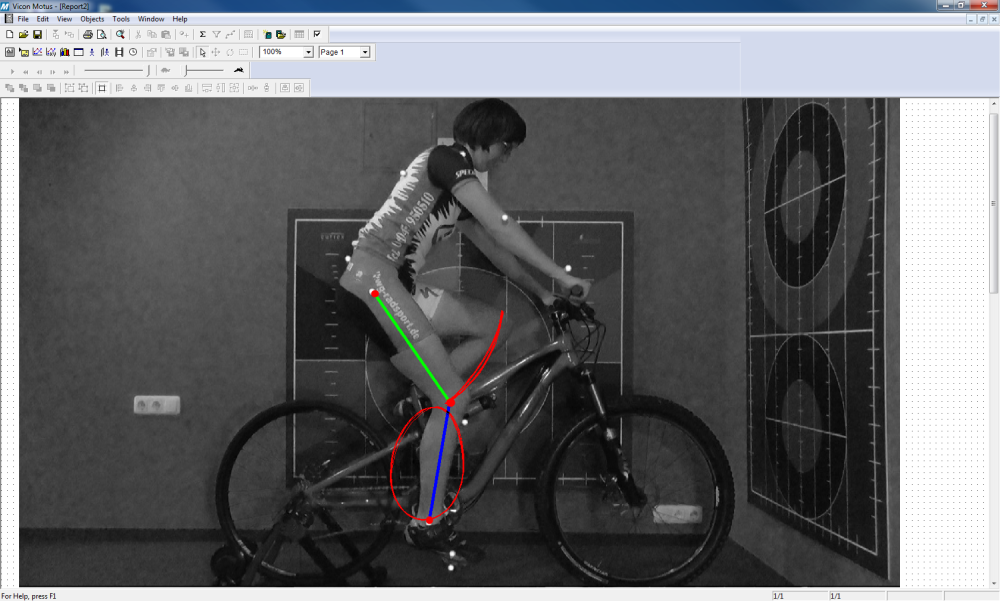## 2D Calculations

The Vicon Motus® 2D module consists of menu-driven software for coordinate scaling, interpolation, kinematic parameter calculations, filtering, and multi-trial averaging. Two-dimensional motion measurement is appropriate for teaching principles and for generating planar angle and displacement measurements for activities, such as running (sagittal, frontal or coronal), lifting (without rotation) and numerous industrial and biological activities.

• 2D coordinate scaling: Accurately scale computer pixels to desired units
• 2D calibration: Use a calibration panel for calculating real distances and other values
• 2D kinematic parameter calculations: Virtual points, centre of mass, linear accelerations, linear displacements, linear velocities, angular velocities, angular accelerations, joint angles, segmental angels, customized angles
• Data filtering: Butterworth Algorithm, Fast Fourier Transform, Cubic Spline, Quintic Spline
• Interpolation: Eliminate any “Black Box” approach to data collection
• Trial averaging: Combining data from multiple trials of the same subject
• Temporal expansion: Expand the frequency up to double the rate at which the data were originally digitized using Shannon’s Sampling Theorem